Varsity Tutors always has a different Complex Analysis Question of the Day ready at your disposal! If you’re just looking to get a quick review into your busy day, our Complex Analysis Question of the Day is the perfect option. Answer enough of our Complex Analysis Question of the Day problems and you’ll be ready to ace the next test. Check out what today’s Complex Analysis Question of the Day is below.

### Question of the Day: Complex Analysis

Cauchy's Residue Theorem is as follows:

Letbe a simple closed contour, described positively. If a functionis analytic insideexcept for a finite number of singular points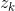inside, then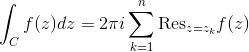For the following problem, use a modified version of the theorem which goes as follows:

Residue Theorem

If a functionis analytic everywhere in the finite plane except for a finite number of singular points interior to a positively oriented simple closed contour, then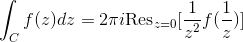Brown, J. W., & Churchill, R. V. (2009). Complex variables and applications. Boston, MA: McGraw-Hill Higher Education.

Use the Residue Theorem to evaluate the integral of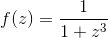in the region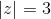.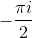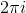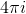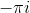### All Complex Analysis Resources